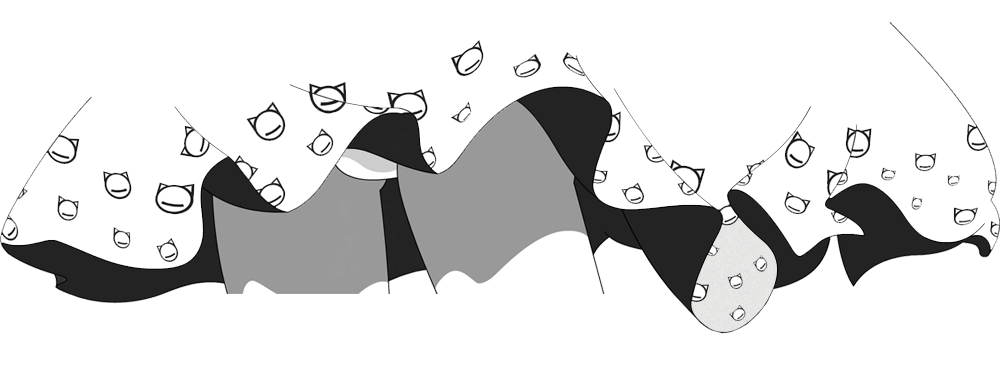# 感知机原理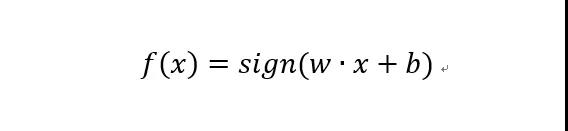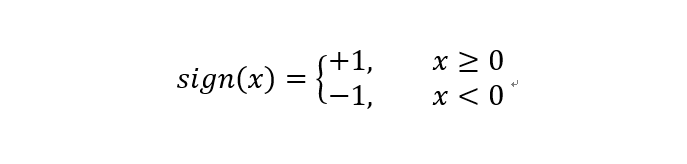w和b为感知机模型参数，也是感知机要学习的东西。w和b构成的线性方程wx+b=0极为线性分离超平面。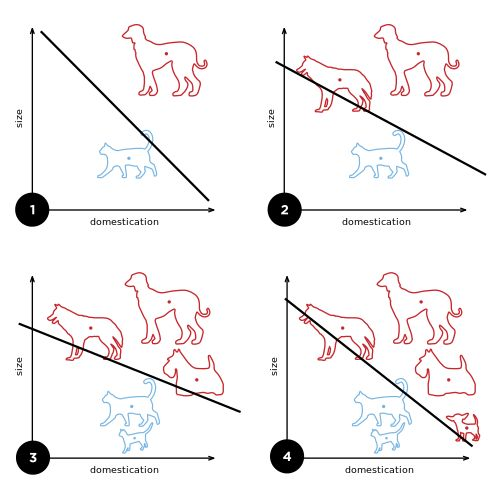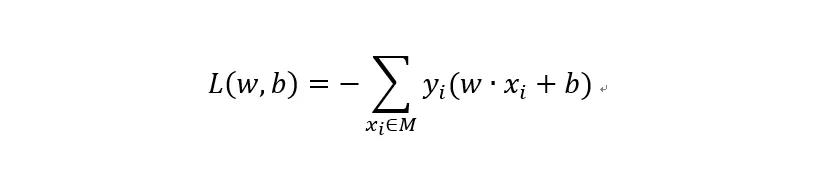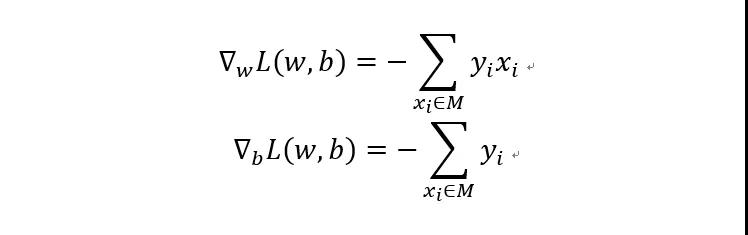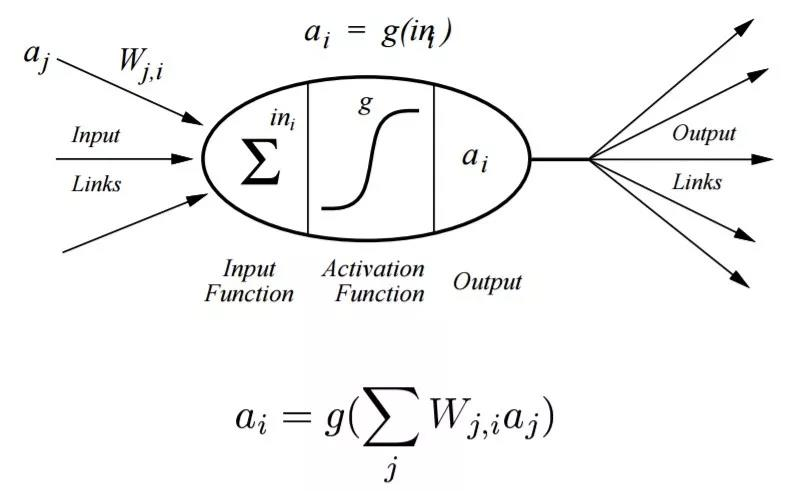# 代码实现

import copy
from matplotlib import pyplot as plt
from matplotlib import animation

training_set = [[(1, 2), 1], [(2, 3), 1], [(3, 1), -1], [(4, 2), -1]]  # 训练数据集
w = [0, 0]  # 参数初始化
b = 0
history = []  # 用来记录每次更新过后的w,b

def update(item):
"""
随机梯度下降更新参数
:param item: 参数是分类错误的点
:return: nothing 无返回值
"""
global w, b, history  # 把w, b, history声明为全局变量
w += 1 * item * item  # 根据误分类点更新参数,这里学习效率设为1
w += 1 * item * item
b += 1 * item
history.append([copy.copy(w), b])  # 将每次更新过后的w,b记录在history数组中

def cal(item):
"""
计算item到超平面的距离,输出yi(w*xi+b)
（我们要根据这个结果来判断一个点是否被分类错了。如果yi(w*xi+b)>0,则分类错了）
:param item:
:return:
"""
res = 0
for i in range(len(item)):  # 迭代item的每个坐标，对于本文数据则有两个坐标x1和x2
res += item[i] * w[i]
res += b
res *= item  # 这里是乘以公式中的yi
return res

def check():
"""
检查超平面是否已将样本正确分类
:return: true如果已正确分类则返回True
"""
flag = False
for item in training_set:
if cal(item) <= 0:  # 如果有分类错误的
flag = True  # 将flag设为True
update(item)  # 用误分类点更新参数
if not flag:  # 如果没有分类错误的点了
print("最终结果: w: " + str(w) + "b: " + str(b))  # 输出达到正确结果时参数的值
return flag  # 如果已正确分类则返回True,否则返回False

if __name__ == "__main__":
for i in range(1000):  # 迭代1000遍
if not check(): break  # 如果已正确分类，则结束迭代
# 以下代码是将迭代过程可视化
# 首先建立我们想要做成动画的图像figure, 坐标轴axis,和plot element
fig = plt.figure()
ax = plt.axes(xlim=(0, 2), ylim=(-2, 2))
line, = ax.plot([], [], 'g', lw=2)  # 画一条线
label = ax.text([], [], '')

def init():
line.set_data([], [])
x, y, x_, y_ = [], [], [], []
for p in training_set:
if p > 0:
x.append(p)  # 存放yi=1的点的x1坐标
y.append(p)  # 存放yi=1的点的x2坐标
else:
x_.append(p)  # 存放yi=-1的点的x1坐标
y_.append(p)  # 存放yi=-1的点的x2坐标
plt.plot(x, y, 'bo', x_, y_, 'rx')  # 在图里yi=1的点用点表示，yi=-1的点用叉表示
plt.axis([-6, 6, -6, 6])  # 横纵坐标上下限
plt.grid(True)  # 显示网格
plt.xlabel('x1')  # 这里我修改了原文表示
plt.ylabel('x2')  # 为了和原理中表达方式一致，横纵坐标应该是x1,x2
plt.title('Perceptron Algorithm (www.hankcs.com)')  # 给图一个标题：感知机算法
return line, label

def animate(i):
global history, ax, line, label
w = history[i]
b = history[i]
if w == 0: return line, label
# 因为图中坐标上下限为-6~6，所以我们在横坐标为-7和7的两个点之间画一条线就够了，这里代码中的xi,yi其实是原理中的x1,x2
x1 = -7
y1 = -(b + w * x1) / w
x2 = 7
y2 = -(b + w * x2) / w
line.set_data([x1, x2], [y1, y2])  # 设置线的两个点
x1 = 0
y1 = -(b + w * x1) / w
label.set_text(history[i])
label.set_position([x1, y1])
return line, label

print("参数w,b更新过程：", history)
anim = animation.FuncAnimation(fig, animate, init_func=init, frames=len(history), interval=1000, repeat=True,
blit=True)
plt.show()